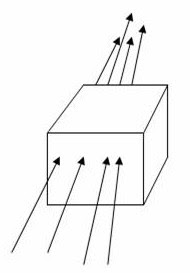## Pages

`“Life is like riding a bicycle.  To keep your balance you must keep moving.”–Albert Einstein`

## Friday, December 24, 2010

### AP Physics C - Multiple Choice Practice Questions involving Electric Flux and Gauss’s Law

Gauss’s law (Gauss theorem) in electrostatics is a very useful tool for calculating electrostatic field produced by a system of charges when the system has some symmetry. You might have realized how simple the calculation is if you choose a suitable Gaussian surface. You should definitely remember the statement of Gauss’s law:

“Electric flux through any closed surface S = Q0 where Q is the total charge enclosed by the surface S and ε0 is the permittivity of free space.”

Today we will discuss a few multiple choice practice questions involving electric flux and Gauss’s law:

(1) A closed cubical surface of side a contains an electric dipole of moment p. The total electric flux through the cubical surface is

(a) zero

(b) 8a2p

(c) 6a2p

(d) 8p/ε0a

(e) 6p/ε0a

The electric dipole contains positive and negative charges of equal magnitude. Therefore the total charge inside the cubical surface is zero. By Gauss’s law the total electric flux through the cubical surface is zero [Option (a)].

(2) A uniform electric field E given by E = 200 ĵ NC–1 exists in a certain region of space. The electric flux through a square of area A = 0.04 î m2 located in this field is (î and ĵ are unit vectors along the x-direction and y-direction respectively)

(a) 5000 Nm2C–1

(b) 5000 Nm2C–1

(c) 8 Nm2C–1

(d) 8 Nm2C–1

(e) zero

The area vector by convention is perpendicular to the plane of the area. Since the area vector is directed along the x-axis, you know that the square lies in the yz plane. Therefore, there is no electric flux passing through the square and the correct option is (e).

[In vector notation electric flux is the scalar product (dot product) of E and A. Therefore we have flux Ф = E.A = 200 ĵ . 0.04 î = 0].(3) A cubical closed surface has N inward electric flux lines passing through one face and N outward electric flux lines passing through the opposite face as shown in the adjoining figure. The flux lines exhibit convergence and there are no flux lines passing through any other face. If the magnitude of the electric field at the centre of the cube is E, the total charge inside the cube is

(a) ε0N

(b) – ε0N

(c) 2ε0N

(d) – 2ε0N

(e) zero

Since the inward flux and the outward flux have the same value, the net flux through the closed surface is zero. By Gauss’s law, we have

0 = q0 where q is the total charge inside the closed surface.

Therefore q = 0.(4) A point positive charge Q is placed at the centre of a cubical closed surface. The outward electric flux through one pair of opposite faces of the cube is

(a) zero

(b) Q0

(c) 2Q0

(d) Q/2ε0

(e) Q/3ε0

The total electric flux lines originating from the positive charge Q is Q0. Since these flux lines are distributed equally among the six faces (or three pairs of faces) of the cube, the flux through one pair of opposite faces of the cube is Q/3ε0.

(5) A hemispherical bowl of radius R is kept inverted on a horizontal table (so that the mouth of the bowl is in contact with the surface of the table). Assume that the surface of the table coincides with the XZ plane of a right handed Cartesian coordinate system. If a uniform electric field E directed along the negative z-direction exists in the entire region, what is the electric flux through the mouth of the hemispherical bowl?

(a) πR2E

(b) πR2E/3

(c) πR2E/3

(d) πR2E

(e) Zero

As the mouth of the bowl is lying in the XZ plane the electric field E (which is along the negative z-direction) is parallel to the plane of the mouth. The electric flux through the mouth of the bowl is therefore zero.(6) A point charge Q is directly above the centre of a square of side a at distance a/2 from the centre (Fig.). The electric flux through the square is

(a) 2Q0

(b) Q0

(c) Q/2ε0

(d) Q/3ε0

(e) Q/6ε0

You may imagine the charge Q to be at the centre of a cube of side a. The total flux through all the six faces of the cube is Q0 by Gauss’s law. Now think of the square given in the question. Since it makes one face of the cube, the flux through it will be one sixth of the total flux. So the correct option is Q/6ε0.

Now, see some useful multiple choice questions (with solution) in this section here.

#### 1 comment:

1.Question 5 here about the inverted hemisphere, can you please tell me, which paper it is from?